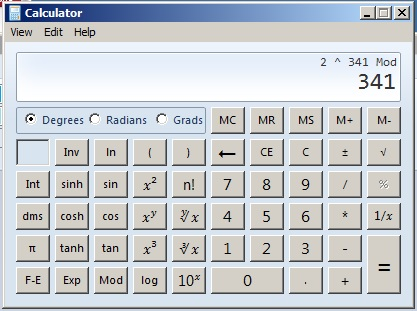# 645 Four Consecutive Odd Numbers Pass a Prime Number Test

There is a quick test to see if an odd number is prime: Plug in the number for x in the equation y = 2^x (mod x). If y = 2, then x is VERY LIKELY a prime number. I call that equation the quick prime number test.

The smallest composite number that gives a false positive to this test is 341. Click on that number to see an in depth description of this quick prime number test and how it relates to Pascal’s triangle.This is only a picture of a calculator.

The second smallest number to give a false positive is 561. Click on that number to see MANY false positives for other tests for that composite number.

645 is not usually tested to see if it is prime because it ends with a 5 and the sum of its digits is a multiple of 3. From those two divisibility rules, we know that 645 is a composite number divisible by both 3 and 5.

Still 645 is the third smallest number to give a false positive to the quick prime number test.

Here is a chart of the quick prime number test applied to the odd numbers from 343 to 681. (A similar chart for odd numbers up to 341 can be seen here.) Whenever y equals 2, I’ve made that 2 red. Prime numbers have been highlighted in yellow. Pseudoprimes 561 and 645 are in bold print.There are 119 numbers less than or equal to 561 that pass this prime number test, and only 3 of those numbers are composite numbers that give a false positive. Amazing.

Perhaps you noticed something else that’s pretty amazing: 641, 643, 645, and 647 are FOUR consecutive odd numbers that pass the quick prime number test, and 645 is the only composite number of the four. Those are the smallest four consecutive odd numbers that pass the test.

It makes me wonder if longer strings of numbers that pass the test are possible. As almost everybody knows 3, 5, and 7 are the only consecutive odd numbers that are also prime numbers. All other strings of three or more consecutive odd numbers contain at least one composite number that is a multiple of 3. Including pseudoprimes like 645 certainly opens up the possibility of longer strings of prime and pseudoprime numbers.

I also continue to be fascinated by the amount of times on the chart that y equals an odd power of 2 (2, 8, 32, 128, 512).

Interesting observation: All three of these pseudoprimes are of the form 4n + 1. All prime numbers of the form 4n + 1 can be written as the sum of two square numbers that have no common factors. 341, 561, and 645 cannot be written as such a sum so they cannot be prime numbers.

______________________________________

645 is the hypotenuse of the Pythagorean triple 387-516-645. What 3-digit number is the greatest common factor of those three numbers?

• 645 is a composite number.
• Prime factorization: 645 = 3 x 5 x 43
• The exponents in the prime factorization are 1, 1, and 1. Adding one to each and multiplying we get (1 + 1)(1 + 1)(1 + 1) = 2 x 2 x 2 = 8. Therefore 645 has exactly 8 factors.
• Factors of 645: 1, 3, 5, 15, 43, 129, 215, 645
• Factor pairs: 645 = 1 x 645, 3 x 215, 5 x 129, or 15 x 43
• 645 has no square factors that allow its square root to be simplified. √645 ≈ 25.39685.